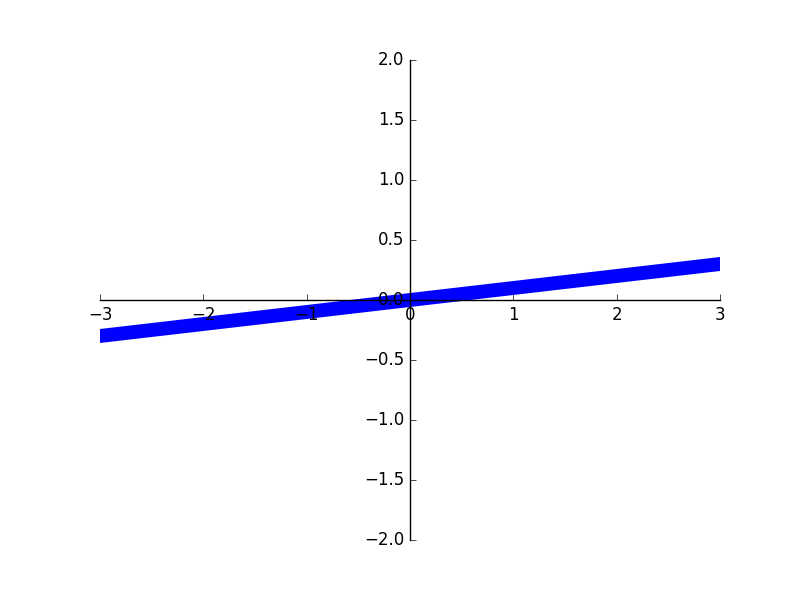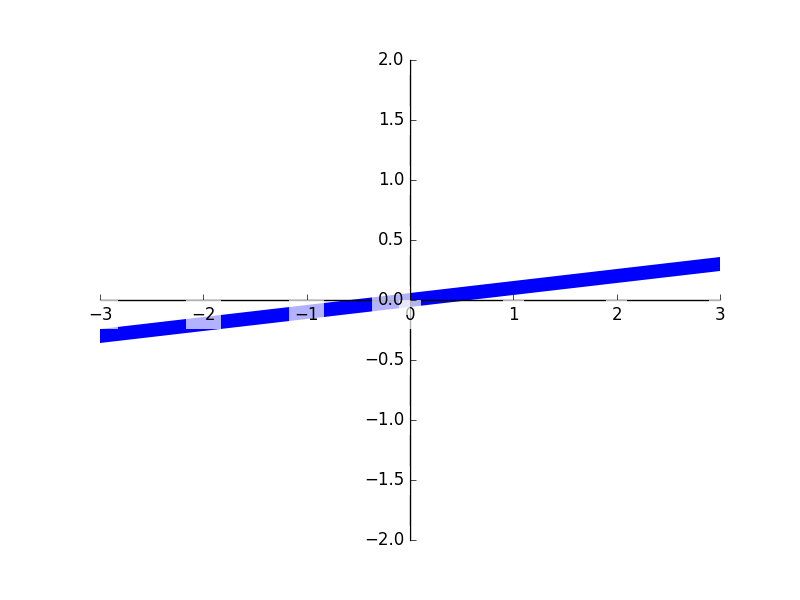# matplotlib tick能见度

632℃

## 生成图形

``````import matplotlib.pyplot as plt
import numpy as np

x = np.linspace(-3, 3, 50)
y = 0.1*x

plt.figure()
# 在 plt 2.0.2 或更高的版本中, 设置 zorder 给 plot 在 z 轴方向排序
plt.plot(x, y, linewidth=10, zorder=1)
plt.ylim(-2, 2)
ax = plt.gca()
ax.spines['right'].set_color('none')
ax.spines['top'].set_color('none')
ax.spines['top'].set_color('none')
ax.xaxis.set_ticks_position('bottom')
ax.spines['bottom'].set_position(('data', 0))
ax.yaxis.set_ticks_position('left')
ax.spines['left'].set_position(('data', 0))``````

[]()

## 调整坐标

``````for label in ax.get_xticklabels() + ax.get_yticklabels():
label.set_fontsize(12)
# 在 plt 2.0.2 或更高的版本中, 设置 zorder 给 plot 在 z 轴方向排序
label.set_bbox(dict(facecolor='white', edgecolor='None', alpha=0.7, zorder=2))
plt.show()``````

[]()